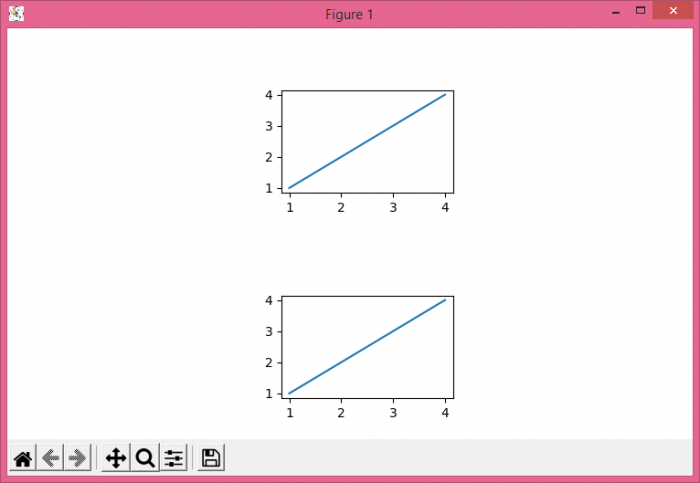# Adjust one subplot's height in absolute way (not relative) in Matplotlib

To adjust one subplot's height in absolute way in Matplotlib, we can take the following steps −

• Set the figure size and adjust the padding between and around the subplots.
• Create a new figure or activate an existing figure.
• For absolute height of subplot, use Axes() class
• Add an axes to the figure.
• Plot the data points on the axes.
• To display the figure, use show() method.

## Example

from matplotlib import pyplot as pl

pl.rcParams["figure.figsize"] = [7.50, 4.50]
pl.rcParams["figure.autolayout"] = True

figure = pl.figure()

axes = pl.Axes(figure, [.4, .6, .25, .25])
pl.plot([1, 2, 3, 4], [1, 2, 3, 4])

axes = pl.Axes(figure, [.4, .1, .25, .25])
pl.show()